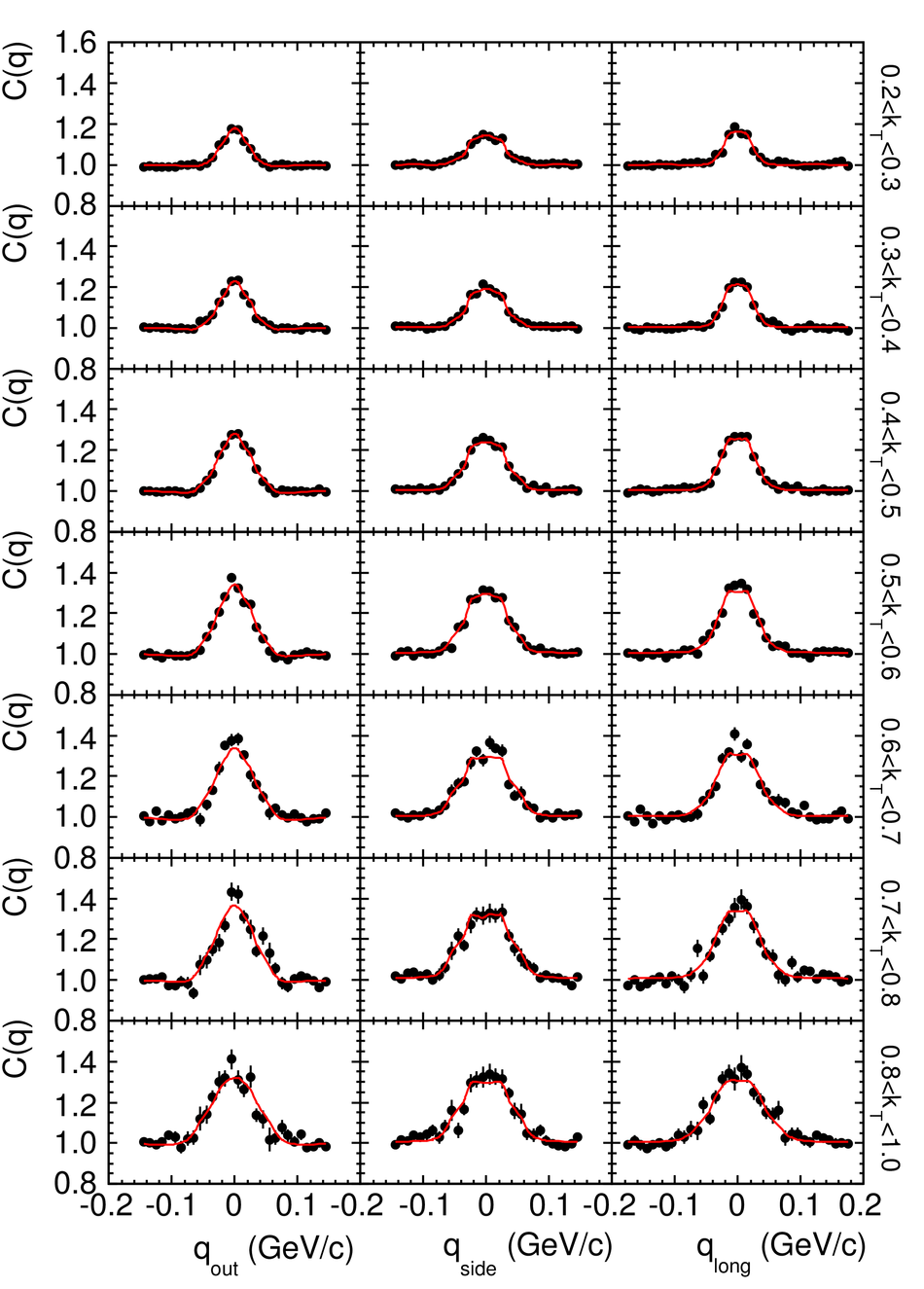# Figure 1

 Projections of the three-dimensional $\pi^-$$\pi^+$ correlation function (points)and of the respective fits (lines) for seven $k_T$ intervals When projecting on one axis the other two components were required to be within (-0.03,0.03) GeV/$c$ The $k_T$ range is indicated on the right-hand side axis in GeV/$c$.